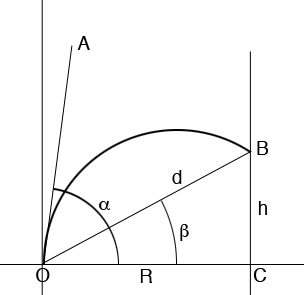SEARCH HOMEMath Central Quandaries & QueriesQuestion from Thys: Hi I have a problem with the formula that i use .(for programming) I have looked all over the web to find a solution but no luck. I have a cannon that shoots a ball at a target I use this formula to calculate what my initial velocity must be to hit the target at a angle of 30 degrees and a distance of 15m (the cannon and target position is known) It works perfectly if both is at same height but if one is higher or lower it misses. In an example I am working with the range is 30m, the angle is 45 degrees and the target is 10m higher than my position. Please help Formula V0 = √[RG / Sin(2α)]Hi Thys,

I found an expression for what you want on the Mechanical Engineering web site at http://www.aboutmech.com/2014/06/equation-of-path-of-projectile.html. It is item number 5. on their page. I don't think however it is in a form that will be particularly useful to you. I redrew the diagram on their site and labeled it to be more consistent with the notation you are usingThe cannon is at $O,$ the target is at $B,$ $d$ is the distance to the target along the line of sight $OB$ and $h$ is the height of the target above the horizontal. The distances are in feet and the angles are measured in degrees. The line segment $OA$ is in the direction of the initial velocity vector of the cannon ball, at an angle of $\alpha$ degrees to the horizontal. With this notation the formula given on the Mechanical Engineering web site is

$d = \frac{u^2}{G \; \cos^2 \beta} \left[ \sin[2(\alpha - \beta) - \beta] - \sin \beta\right] = \frac{u^2}{G \; \cos^2 \beta} \left[ \sin[2 \alpha - 3 \beta)] - \sin \beta\right]$

where $G$ is the force due to gravity ad $u$ is the initial velocity.

Using the facts that

$\sin(2 \alpha - 3 \beta) = \sin 2 \alpha \cos 3 \beta + \cos 2 \alpha \sin 3 beta$

$\sin 3 \beta = 3 \sin \beta - 4 \sin^3 \beta$

$\cos 3 \beta = 4 \cos^3 \beta - 3 \cos \beta$

and reading the values of $\sin \beta$ and $\cos \beta$ from the diagram the expression for $d$ above becomes

$d = \frac{u^2}{G \frac{R^2}{d^2}} \left[ \left( 4 \frac{R^3}{d^3} - 3 \frac{R}{d} \right) \sin 2 \alpha + \left(3 \frac{h}{d} - 4 \frac{h^3}{d^3}\right) \cos 2 \alpha - \frac{h}{d}\right].$

Since $R^2 + h^2 = d^2$ this simplifies to

$1 = \frac{u^2}{G R^2} \left[ \frac{R^3 - 3 R h^2}{R^2 + h^2} \sin 2 \alpha + \frac{3 h R^2 - h^2}{R^2 + h^2} \cos 2 \alpha - h \right]$

or

$u = \sqrt{ \frac{G R^2}{\left[ \frac{R^3 - 3 R h^2}{R^2 + h^2} \sin 2 \alpha + \frac{3 h R^2 - h^2}{R^2 + h^2} \cos 2 \alpha - h \right] }}$

This expression looks onerous but when $h = 0,$ the cannon and the target are at the same altitude it reduces to the expression you sent

$u = \sqrt{\frac{RG}{\sin 2 \alpha}}.$

For the example you sent with $\alpha = 45^o$ you have $\sin 2 \alpha = \sin 90^o = 1,$ and $\cos \alpha = \cos 90^o$ so

$u = \sqrt{ \frac{GR^2 (R^2 + h^2)}{R^3 - 3 R h^2}}$

I hope this helps,
HarleyMath Central is supported by the University of Regina and the Imperial Oil Foundation.